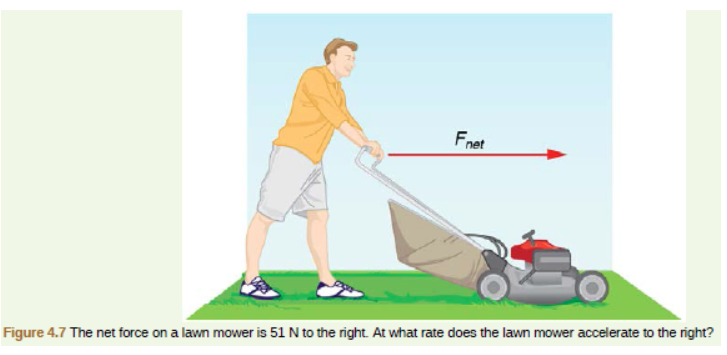# Problem: In Figure 4.7, the net external force on the 24-kg mower is stated to be 51 N. If the force of friction opposing the motion in 24 N, what force F (in newtons) is the person exerting on the mower?

###### FREE Expert Solution

Fnet is to the right.

Applied force, F is to the right.

The friction force, f, is to the left.

81% (299 ratings)###### Problem Details

In Figure 4.7, the net external force on the 24-kg mower is stated to be 51 N. If the force of friction opposing the motion in 24 N, what force F (in newtons) is the person exerting on the mower?